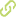# Hyper-heuristics Reversed: Learning to Combine Solvers by Evolving Instances•
• Overview
•
• Identity
•
•
• View All
•

### Abstract

• © 2019 IEEE.It is common to find that training of selection hyper-heuristics is done perturbatively. The process usually starts with a random selection module and iterates over a set of instances until finding appropriate values for such module. In this work, however, we present a model for creating selection hyper-heuristics constructively. To achieve so, we use a set of instances evolved for such a task. For each low-level heuristic, we evolved a set of problem instances that are more easily solvable by that particular heuristic (compared to the other ones). Each group contains instances easily solvable with the corresponding heuristic but not with the remaining ones. Thus, our model creates its selector by calculating the centroid of each group. For doing so, the model defines a rule that maps said centroid to one corresponding action (in this case, a low-level heuristic). To test our approach, we select the one-dimensional Bin Packing Problem and set four target performance levels (which we refer to as deltas) for instance generation. Then, we analyze all the possible combinations of deltas. We study how performance of the generated hyper-heuristics shift when they are created using a different number of instances. Our data shows the feasibility of creating a hyper-heuristic under the stated conditions. Effectiveness of the model depends on the deltas used though we observed that higher deltas are useful while lower deltas are not. For example, when considering a delta level of 2.0, our method produced hyper-heuristics with an accumulated average waste 12% lower than that of the best heuristic. But, for a delta level of 0.5, it became impossible to outperform the heuristics.

• June 1, 2019